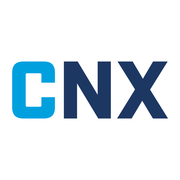2,404 recent views
Shareable Certificate
Earn a Certificate upon completion
100% online
Start instantly and learn at your own schedule.
Intermediate Level

ML workflow knowledge is required, as is experience with Python or similar languages. Basic knowledge of math and statistics is also recommended.

Approx. 20 hours to complete
English

### What you will learn

• Train and evaluate linear regression models.

• Train binary and multi-class classification models.

• Evaluate and tune classification models to improve their performance.

• Train and evaluate clustering models to find useful patterns in unsupervised data.

### Skills you will gain

Machine LearningclusteringclassificationLinear RegressionMachine Learning (ML) Algorithms
Shareable Certificate
Earn a Certificate upon completion
100% online
Start instantly and learn at your own schedule.
Intermediate Level

ML workflow knowledge is required, as is experience with Python or similar languages. Basic knowledge of math and statistics is also recommended.

Approx. 20 hours to complete
English

### Offered byWeek
1

## Week 1

2 hours to complete

## Build Linear Regression Models Using Linear Algebra

2 hours to complete
14 videos (Total 36 min), 2 readings, 1 quiz
14 videos
CAIP Specialization Introduction3m
Build Linear Regression Models Using Linear Algebra Module Introduction1m
Linear Regression1m
Linear Equation2m
Straight Line Fit to Data Example1m
Linear Regression in Machine Learning3m
Matrices in Linear Regression4m
Normal Equation5m
Cost Function1m
MSE and MAE3m
Coefficient of Determination1m
Normal Equation Shortcomings43s
Overview2m
Guidelines for Building a Regression Model Using Linear Algebra10m
1 practice exercise
Building Linear Regression Models Using Linear Algebra30m
Week
2

## Week 2

3 hours to complete

## Build Regularized and Iterative Linear Regression Models

3 hours to complete
8 videos (Total 14 min), 3 readings, 1 quiz
8 videos
Regularization Techniques59s
Ridge Regression2m
Lasso Regression1m
Elastic Net Regression2m
Iterative Models1m
Overview2m
Guidelines for Building a Regularized Linear Regression Model5m
Guidelines for Building an Iterative Linear Regression Model5m
1 practice exercise
Building Regularized and Iterative Linear Regression Models30m
Week
3

## Week 3

3 hours to complete

## Train Classification Models

3 hours to complete
9 videos (Total 13 min), 3 readings, 1 quiz
9 videos
Linear Regression Shortcomings1m
Logistic Regression1m
Decision Boundary51s
Cost Function for Logistic Regression1m
k-Nearest Neighbor (k-NN)2m
Logistic Regression vs. k-NN35s
Multi-Label and Multi-Class Classification1m
Multinomial Logistic Regression2m
Overview2m
Guidelines for Training Binary Classification Models10m
Guidelines for Training Multi-Class Classification Models3m
1 practice exercise
Training Classification Models30m
Week
4

## Week 4

3 hours to complete

## Evaluate and Tune Classification Models

3 hours to complete
16 videos (Total 27 min), 3 readings, 1 quiz
16 videos
Model Performance1m
Confusion Matrix1m
Classifier Performance Measurement58s
Accuracy1m
Precision1m
Recall1m
F₁ Score1m
Thresholds and AUC2m
Precision–Recall Curve (PRC)1m
Hyperparameter Optimization53s
Grid Search2m
Randomized Search1m
Bayesian Optimization2m
Genetic Algorithms2m